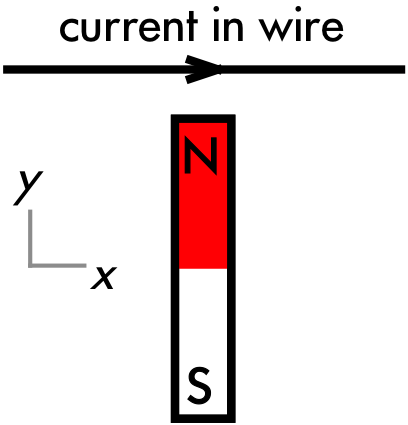## 20180410

### Physics quiz question: magnetic force of magnet on current-carrying wire

Physics 205B Quiz 6, spring semester 2015
Cuesta College, San Luis Obispo, CAThe direction of the force on the current-carrying wire near the north end of this magnet is:
(A) up ↑.
(B) down ↓.
(C) left ←.
(D) right →.
(E) into the page ⊗.
(F) out of the page ⊙.
(G) (Some other (diagonal) direction not listed above.)
(H) (No direction, as the magnetic force is zero.)

Correct answer (highlight to unhide): (F)

For the field model of magnetism, the source object is the magnet, which generates a magnetic field around itself. This magnetic field (generated by the magnet) then exerts a force on the test object: the flow of positive charges of the current in the wire.

At the position of the wire, the magnetic field just above the north end of the magnet points up along the plane of the page, as magnetic field lines come out of the north end of magnets (while magnetic field lines go into the south end of magnets).

Using the first right-hand rule (RHR1), where the thumb points along the direction of the current in the wire, which is the velocity direction v of positive charge flow: to the right in the plane of the page; the index finger along the direction of the magnetic field B: up along the plane of the page; the middle finger then must point perpendicularly out of the page of the page, which is the direction of the force F on the flow of positive charges for the current in the wire.

Sections 30882, 30883
Exam code: quiz06t3SL
(A) : 6 students
(B) : 3 students
(C) : 4 students
(D) : 1 student
(E) : 12 students
(F) : 18 students
(G) : 2 students
(H) : 0 students

Success level: 34%
Discrimination index (Aubrecht & Aubrecht, 1983): 0.62